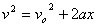Page one derivations X = the distance an object travels t = elapsed time to travel distance X Vo = velocity at X = 0, t = 0 V = velocity at end of time and distance traveled a = acceleration, assumed to be unchangiing during t We define acceleration as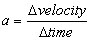define Dvelocity ..............DtimeRearrange terms to get page 1 line 1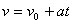We take as axiom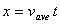Define V ave = 1/2 (Vo +V) This is page 2, line 2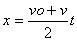Start with p1,l2.......For V sub in p1,l1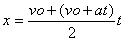Rearrange terms to get page 1 line 3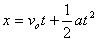Take eq p1, l1 and square it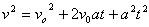Factor this line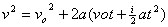Terms in ( ) = x......This is page 1, line 4